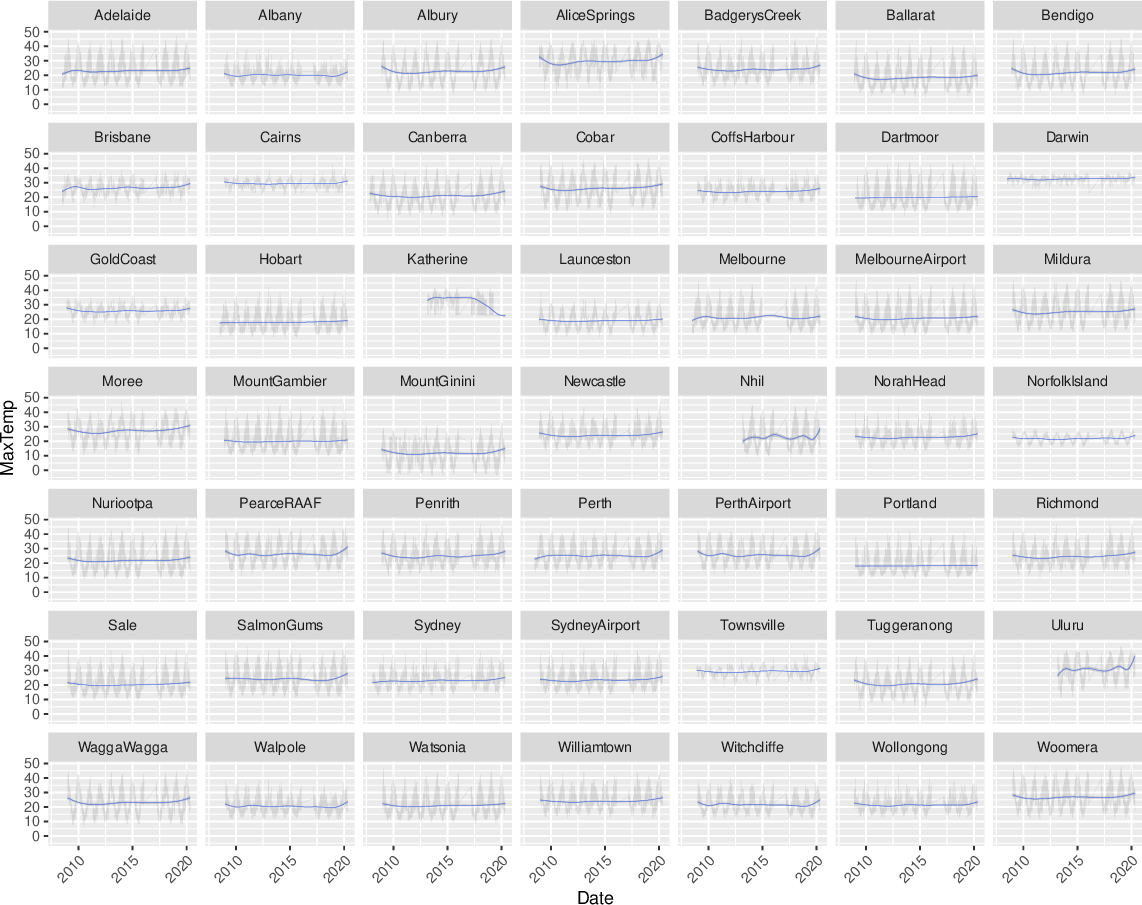Data Science Desktop Survival Guide by Graham WilliamsDesktop Survival Project Home Preface Data Science Introducing R R Constructs R Tasks R Strings R Read, Write, and Create Data Template Data Exploration Data Wrangling Data Visualisation Statistics ML Template ML Scenarios ML Activities ML Applications ML Algorithms Cluster Analysis Decision Trees Computer Vision Graph Data Privacy Literate Data Science Coding with Style Resources Bibliography Index

## Faceted Location Thin Lines

20200608ds %>%   ggplot(aes(x=date, y=max_temp)) +   geom_line(alpha=0.1, size=0.05) +   geom_smooth(method="gam", formula=y~s(x, bs="cs"), size=0.05) +   facet_wrap(~location) +   theme(axis.text.x=element_text(angle=45, hjust=1)) +   labs(x=vnames["date"], y=vnames["max_temp"]) An alternative is to present the plot as a line chart rather than a scatter plot. It does make more sense for a time series plot such as this, though the effect is little changed due to the amount of data being displayed. Changing to lines simply uses ggplot2::geom_line() instead of ggplot2::geom_point(). Very thin lines are used as specified through the size= option. Nonetheless, the data remains quite dense.